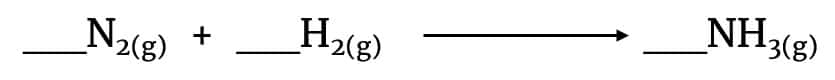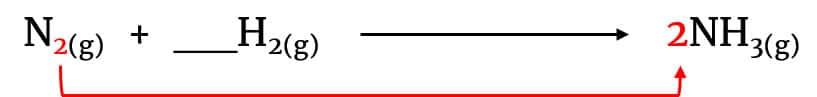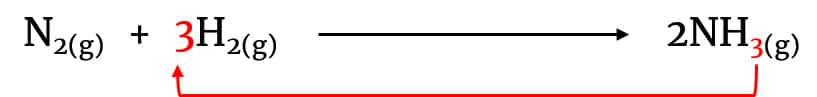# Easy way to balance chemical equations for GCSE

## Balancing chemical equations

A chemical equation balancing question is normally worth 1 or 2 marks, which means you have at most 2 minutes to solve it. Everyone is taught the table method when learning to balance chemical equations (where you use a table to track the numbers of each element on each side of the equation) but you really don’t have time to be constructing tables in an exam.

Instead, I recommend trying the subscript method to balance chemical equations first. The idea behind this is simple. You cannot change subscripts in chemical formula, so instead use them to help balance the equation. This works because in the majority of equations, an element’s subscript on one side of the equation will be its balancing number (or coefficient) on the other side.

You can remember this method as a simple rule: use the subscripts as balancing numbers.

Here’s an example of how this works using the Haber process equation.This is obviously unbalanced currently because we have 2 nitrogen atoms on the left-hand side and only 1 on the right-hand side, and 2 hydrogen atoms on the left-hand side but 3 on the right-hand side.

Since we can’t change the subscripts, start by using nitrogen’s subscript from the left-hand side as its coefficient on the right-hand side:Then do the same with hydrogen’s subscript on the right-hand side, using it to balance hydrogen on the left-hand side. This now balances the equation.## How useful is this method?

Having looked through GCSE chemistry papers from AQA, CIE, Edexcel and OCR, I estimate this method is useful in balancing about 95% of chemical equations at GCSE level.

For some harder equations, often the subscript method is the starting point but you’ll have to use other rules to complete the balancing. (I wrote a longer post about the balancing chemical equations that you might want to check out).

## 25 balancing chemical equations questions from GCSE papers

In no particular order of difficulty, here are 25 questions on balancing chemical equations, taken from various exam boards’ past papers. The solutions are at the bottom.

### AQA GCSE

1. __CaCO3 + __HCl  →  __CaCl2  + __CO2  +  __H2O

(Paper C1H, June 2012)

1. __Fe2O3 +  __C  → __Fe  +  __CO2

(Paper C1H, January 2012 and 9-1 Paper 1F specimen)

1. __C2H5OH + __O2  →  __CO2  +  __H2O

(Paper C3H Specimen)

1. __N2 +  __H2  →  __NH3

(9-1 Paper 2F specimen)

### CIE IGCSE

1. __Zn +  __HCl  →  __ZnCl2  + __H2

(Summer 2003, Paper 2)

1. _SiO2 +  __C →  __Si  +  __CO

(Summer 2004, Paper 3)

1. __Fe(OH)2 +  __O2  +  __H2O →  __Fe(OH)3

(Summer 2010, Paper 3 Variant 2)

1. __PCl3 +  __H2O  →  __HCl  +  __H3PO3

(Summer 2011, Paper 3 Variant 2)

1. __Fe2O3 +  __CO  →  __Fe  +  __CO2

(Summer 2017, Paper 3 Variant 3)

1. __NH3 +  __O2  →  __NO  + __H2O

(Summer 2017, Paper 3 Variant 3)

1. __C5H11 +  __O2  →  __CO2  + __H2O

(Summer 2018, Paper 3 Variant 1)

1. __NO2 +  __NaOH  →  __NaNO3  +  __NaNO2  +  __H2O

(Summer 2018, Paper 3 Variant 2)

1. __CoO +  __O2  →  __Co3O4

(Summer 2018, Paper 3 Variant 2)

### Edexcel GCSE and IGCSE

1. __NH3 +  __H2O2  →  __N2H4  +  __H2O

(IGCSE Paper 1CR, June 2014)

1. __H2O2 →  __H2O  +  __O2

(IGCSE Paper 1C, January 2015)

1. __C3H8 +  __O2  →  __CO2  +  __H2O

(IGCSE Paper 1C, January 2015)

1. __CuO +  __HCl  →  __CuCl2  +  __H2O

(GCSE Paper 1C, November 2012)

1. __Cu +  __O2  →  __CuO

(IGCSE Paper 1C, March 2013)

1. __CH4 +  __O2  →  __CO  +  __H2O

(IGCSE Paper 1C, March 2013)

1. __NdF3 + __Ca  →  __Nd  +  __CaF2

(IGCSE Paper 1CR, May 2016)

1. __CH4 +  __H2O  →  __CO  + __H2

(IGCSE Paper 1CR, May 2016)

1. __NO2 + __O2  + __ H2O  →  __HNO3

(IGCSE Paper 1C, January 2013)

1. __HCl + __MnO2  →  __MnCl2  +  __H2O  +  __Cl2

(IGCSE Paper 1CR, May 2013)

### OCR GCSE

1. __NO2 + __H2O  →  __HNO2  +  __HNO3

(Paper 1F, June 2014)

1. __C6H14 + __O2  →  __CO2  +  __H2O

(Paper 1H, June 2015)

1. CaCO3 + 2HCl  →  CaCl2  + CO2  +  H2O
2. 2Fe2O3 +  3C  → 4Fe  +  3CO2
3. C2H5OH + 3O2  →  2CO2  +  3H2O
4. N2 +  3H2  →  2NH3
5. Zn +  2HCl  →  ZnCl2  + H2
6. SiO2 +  2C →  Si  +  2CO
7. 2Fe(OH)2 +  ½ O2  +  H2O →  2Fe(OH)3
8. PCl3 +  3H2O  →  3HCl  +  H3PO3
9. Fe2O3 +  3CO  →  2Fe  +  CO2
10. 4NH3 +  5O2  →  4NO  + 6H2O
11. C5H11 +  8 O2  →  5CO2  + 6H2O
12. 2NO2 +  2NaOH  →  NaNO3  +  NaNO2  +  H2O
13. 6CoO +  O2  →  2Co3O4
14. 2NH3 +  H2O2  →  N2H4  +  2H2O
15. H2O2 →  H2O  +  ½ O2
16. C3H8 +  5O2  →  3CO2  +  4H2O
17. CuO +  2HCl  →  CuCl2  +  H2O
18. 2Cu +  O2  →  2CuO
19. 2CH4 +  2O2  →  2CO  +  2H2O
20. 2NdF3 + 3Ca  →  2Nd  +  3CaF2
21. CH4 +  H2O  →  CO  + 3H2
22. 2NO2 + ½ O2 + H2O →  2HNO3
23. 4HCl + MnO2  →  MnCl2  +  2H2O  +  Cl2
24. 2NO2 + H2O  →  HNO2  +  HNO3
25. 2C6H14 + 19O2  →  12CO2  +  14H2O